• 1、什么是指数分布族 1.1 基本描述 指数型分布是一类重要分布族，在统计推断中，指数型分布族占有重要地位，在各领域应用广泛。许多统计分布都是指数型分布，彼此之间具有一定共性，在研究其统计性质与...
1、什么是指数分布族
1.1 基本描述
指数型分布是一类重要的分布族，在统计推断中，指数型分布族占有重要的地位，在各领域应用广泛。许多的统计分布都是指数型分布，彼此之间具有一定的共性，在研究其统计性质与分布特征时，利用指数型分布族的特征，可以将这一族分布的特征分别表示出。在广义线性模型的统计推断中，常假设样本服从指数型分布。
1.2 定义
指数分布族可以写成如下的形式：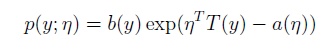在这里，η叫做分布的自然参数，a(η)叫做累积量母函数（又称log partition function）。exp(-α(η))这个量是分布p(y;η)的归一化常数，用来确保分布p(y;η)对y的积分为1。T(y)称为充分统计量（sufficient statistic）,对于我们考虑的分布，一般认为T(y)=y。
一组确定的T，a和b定义了这样一个以η为参数的分布族。对于不同的η，我们可以得到指数分布族中不同的分布。
1.3 数学特征
对于单参数指数型分布的随机变量，记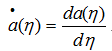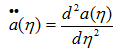，分别表示关于η的函数a对η求一二阶导数，则有以下结论：
指数型分布随机变量的期望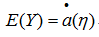指数型分布随机变量的方差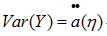2、高斯分布属于指数分布族的证明
对于高斯分布，当方差已知时，（方差对模型的参数没有影响，所以我们可以任意地选一个方差），在这里我们令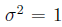，则其分布可以表示为：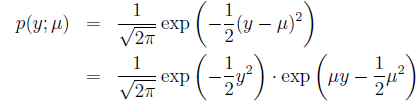为了将其向指数分布族靠拢，我们进行如下表示：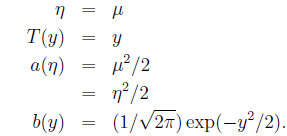这显示了高斯分布可以被写成是指数分布族的形式，所以高斯分布属于指数分布族。
进一步地，我们用指数分布族的性质去验证一下，有：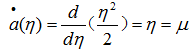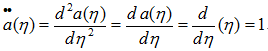刚好是高斯分布的期望和方差，所以验证成功。

3、二项分布属于指数分布族的证明
对于二项分布（伯努利分布），每一个取不同均值的参数Φ，就会唯一确定一个y属于{0,1}之间的分布。所以可以表示为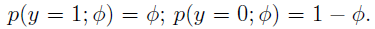故二项分布的分布函数只以Φ作为参数，统一这样表示二项分布：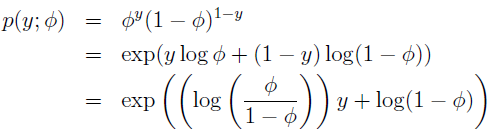这样，自然参数为：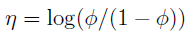，翻转一下，有：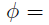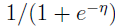为了进一步将二项分布向指数分布族靠拢，我们可以进行如下表示：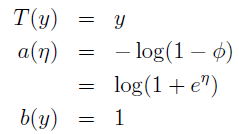这显示了二项分布可以被写成是指数分布族的形式，所以二项分布属于指数分布族。

进一步地，我们用指数分布族的性质去验证一下，有：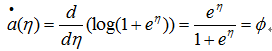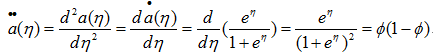刚好是二项分布的期望与方差，故满足性质。
转载于:https://www.cnblogs.com/linyuanzhou/p/4947931.html
展开全文• 指数族是一类分布，包括高斯分布、伯努利分布、二项分布、泊松分布、Beta 分布、Dirichlet 分布、Gamma 分布等一系列分布。指数族分布可以写为统一形式： p(x∣η)=h(x)exp(ηTϕ(x)−A(η))=1exp(A(η))h(x)exp...
指数族分布
指数族是一类分布，包括高斯分布、伯努利分布、二项分布、泊松分布、Beta 分布、Dirichlet 分布、Gamma 分布等一系列分布。指数族分布可以写为统一的形式：
$p(x|\eta) = h(x)exp(\eta^T \phi(x) - A(\eta)) = \frac{1}{exp(A(\eta))} h(x)exp(\eta^T \phi(x))$
其中， $\eta$ 是参数向量，$A(\eta)$是对数配分函数（归一化因子），$\phi(x)$叫做充分统计量，包含样本集合所有的信息，例如高斯分布中的均值和方差。充分统计量常用于在线学习中，对于一个数据集，只需要记录样本的充分统计量即可。
对于一个模型分布假设（似然函数），那么我们在求解对数似然函数时，指数族分布的对数似然函数能给计算带来很大的便利。
对于一个包含$N$个样本的数据集$X={(x_1,x_2, \dots,x_N)}$，指数族分布的对数似然函数求解形式为：
\begin{aligned} \underset {\eta}{\operatorname {arg\,max} } \, log p(X|\eta) &= \underset {\eta}{\operatorname {arg\,max} } \, log \prod_{i=1}^N p(x_i | \eta) \\ &= \underset {\eta}{\operatorname {arg\,max} } \, log \left\{ \left[ \prod_{i=1}^N h(x_i) \right] \left[ exp \left( \eta^T \sum_{i=1}^N \phi(x_i) \right) \right] \left[ N A(\eta) \right] \right\} \end{aligned}
由于我们是对$\eta$做最大化，因此式中与$\eta$无关的系数均视为常量，这样上式可以简化为：
\begin{aligned} L &= \underset {\eta}{\operatorname {arg\,max} } \, log \left\{ \left[ \prod_{i=1}^N h(x_i) \right] \left[ exp \left( \eta^T \sum_{i=1}^N \phi(x_i) \right) \right] - \left[ N A(\eta) \right] \right\} \\ &= \underset {\eta}{\operatorname {arg\,max} } \, \left\{ \left[ exp \left( \eta^T \sum_{i=1}^N \phi(x_i) \right) \right] - \left[ N A(\eta) \right] \right\} \end{aligned}
对数似然函数对$\eta$求导的时候可以发现它简化了运算，求导结果如下：
$\frac{\partial L}{\partial \eta} = \sum_{i=1}^N \phi(x_i) - N A^{'}(\eta) = 0：$
$A^{'}(\eta) = \sum_{i=1}^N \frac{\phi(x_i)}{N}$
这样可以发现，只需要将对数配分函数$A^{'}(\eta)$对$\eta$求倒数就等于所有的充分统计量$\phi(x_i)$的累计和，的确大大的简化了运算。
一维高斯分布的指数族形式
以一维高斯分布为例，高斯分布函数可以写成：
$p(x|\theta) = \frac{1}{\sqrt{2 \pi} \sigma} exp\left( -\frac{ (x - \mu)^2 }{2 \sigma^2} \right)$
将这个式子改写为：
\begin{aligned} \frac{1}{\sqrt{2 \pi} \sigma} exp\left( - \frac{ 1 }{2 \sigma^2} (x^2 -2 \mu x + \mu^2) \right) &= exp\left(log(2 \pi \sigma^2)^{-1/2} \right) exp\left(- \frac{ 1 }{2 \sigma^2} (-2 \mu \quad 1 ) \left( \begin{array}{c} & x \\ x^2 \end{array} \right) -\frac{\mu^2}{2 \sigma^2} \right)\\ &= exp \left\{ \left( \frac{\mu}{\sigma^2},-\frac{1}{2\sigma^2} \right) \left( \begin{array}{c} x \\ x^2 \end{array} \right) - \left( \frac{\mu^2}{2 \sigma^2} + \frac{1}{2}log 2\pi \sigma^2 \right) \right\} \end{aligned}
因此，我们可以得到$\eta^T = \left(\frac{\mu}{\sigma^2},-\frac{1}{2\sigma^2} \right)$，$\phi(x) = \left( \begin{array}{c} x \\ x^2 \end{array}\right)$，$A(\eta) = \left(\frac{\mu^2}{2 \sigma^2} + \frac{1}{2}log (2\pi \sigma^2) \right)$，将$\eta^T$写成下式形式：
$\eta = \left( \begin{array}{c} \frac{\mu}{\sigma^2}\\ -\frac{1}{2 \sigma^2} \end{array}\right)=\left( \begin{array}{c} \eta_1 \\ \eta_2 \end{array}\right)$
可以解得$\sigma^2$，$\mu$与$\eta_1$，$\eta_2$间的关系如下：
\left\{ \begin{aligned} \sigma^2 = -\frac{1}{2 \eta_2} \\ \mu = - \frac{\eta_1}{2 \eta_2} \end{aligned} \right.
于是$A(\eta)$可以表示为：
$A(\eta) = -\frac{\eta_1^2}{4 \eta_2} - \frac{1}{2}log(-\frac{\pi}{\eta_2})$
因此，我们可以将高斯分布的标准指数族分布形式可以写成：
\left\{ \begin{aligned} p(x|\eta) &= exp \left(\eta^T \phi(x) - A(\eta) \right) \\ \eta^T &= \left(\frac{\mu}{\sigma^2},-\frac{1}{2\sigma^2} \right) \\ \phi(x) &= \left( \begin{array}{c} x \\ x^2 \end{array}\right) \\ A(\eta) &= -\frac{\eta_1^2}{4 \eta_2} - \frac{1}{2}log(-\frac{\pi}{\eta_2}) \end{aligned} \right.
高斯分布的极大似然估计解
一元高斯分布的对数似然函数形式如下：
\begin{aligned} logp(X|\theta) &= log \prod_{i=1}^{N} p(x_i | \theta) \\ &= \sum_{i=1}^N logp(x_i|\theta) \\ &= \sum_{i=1}^N log \frac{1}{\sqrt{2x_i} \sigma} exp\left( -\frac{(x - \mu)^2}{2 \sigma^2}\right) \\ &= \sum_{i=1}^N \left[ log \frac{1}{\sqrt 2 \pi } +log \frac{1}{\sigma} - \frac{(x - \mu)^2}{2 \sigma^2} \right] \end{aligned}
使用极大似然估计对其进行求解：
\begin{aligned} logp(X|\theta) &= log \prod_{i=1}^{N} p(x_i | \theta) \\ &= \sum_{i=1}^N logp(x_i|\theta) \\ &= \sum_{i=1}^N log \frac{1}{\sqrt{2x_i} \sigma} exp\left( -\frac{(x - \mu)^2}{2 \sigma^2}\right) \\ &= \sum_{i=1}^N \left[ log \frac{1}{\sqrt 2 \pi } +log \frac{1}{\sigma} - \frac{(x - \mu)^2}{2 \sigma^2} \right] \end{aligned}
对$\mu$求偏导可得：
$\frac{\partial}{\partial \mu} \sum_{i=1}^N (x_i - \mu)^2 = \sum_{i=1}^N 2(x_i - \mu)(-1) = 0 \\ \Rightarrow \mu_{MLE} = \frac{1}{N} \sum_{i=1}^N x_i$
接着求解$\sigma^2$的极大似然估计：
\begin{aligned} \sigma^2_{MLE} &= \underset {\sigma}{\operatorname {arg\,max\,log}} \, p(X|\theta)\\ &= \underset {\sigma}{\operatorname {arg\,max}}\, (-log\sigma - \frac{1}{2 \sigma^2}(x_i-\mu)^2) \\ \Rightarrow \frac{\partial}{\partial \sigma} &= \sum_{i=1}^{N} \left[ -\frac{1}{\sigma} +(x_i - \mu) \sigma^{-3} \right] = 0 \\ \Rightarrow \sigma^2_{MLE} &= \frac{1}{N} \sum_{i=1}^{N}(x_i - \mu_{MLE})^2 \end{aligned}
由极大似然估计可以求出一维高斯分布的参数估计值，能够发现这是很繁琐的步骤，需要对每个参数都进行一次参数估计，所以接下来将高斯分布写作指数族分布的形式，我们来看看指数族分布是如何简化计算的。
高斯分布的指数族形式参数估计
将上述高斯分布写成指数族分布的形式：
\begin{aligned} p(X|\eta) = exp \left[ \eta^T \phi(x) - \left( \frac{\eta_1^2}{4 \eta_2} - \frac{1}{2}log(-\frac{\pi}{\eta_2}) \right) \right] \end{aligned}
\left\{\begin{aligned} \eta^T &= \left( \begin{array}{c} \frac{\mu}{\sigma^2} \\ -\frac{1}{2\sigma^2} \end{array} \right) \\ \phi(x) &= \left( \begin{array}{c} x \\ x^2 \end{array}\right) \\ A(\eta) &= -\frac{\eta_1^2}{4 \eta_2} - \frac{1}{2}log(-\frac{\pi}{\eta_2})\end{aligned}\right.
通过第一节中求出的关系：
$A^{'}(\eta_{MLE}) = \sum_{i=1}^{N} \frac{\phi(x_i)}{N}$
对指数族分布的高斯分布进行参数估计：
$\left[ \begin{array}{c} \frac{\partial A(\eta)}{\partial \eta_1} \\ \frac{\partial A(\eta)}{\partial \eta_2} \end{array} = \right] \left[ \begin{array}{c} -\frac{\eta_1}{2 \eta_2} \\ \frac{\eta_1^2}{4 \eta_2^2} - \frac{1}{2 \eta_2} \end{array} \right] = \left[ \begin{array}{c} \frac{\sum_{i=1}^{N} x_i}{N} \\ \frac{\sum_{i=1}^{N} x_i^2}{N} \end{array} \right]$
这里我们就可以发现上式中的第二部分与均值和方差间的关系：
$\left[ \begin{array}{c} -\frac{\eta_1}{2 \eta_2} \\ \frac{\eta_1^2}{4 \eta_2^2} - \frac{1}{2 \eta_2} \end{array} \right ] = \left[ \begin{array}{c} \mu \\ \mu^2 + \sigma^2 \end{array} \right]$
因此，通过对指数族分布的高斯分布参数估计可以得到：
$\hat{\mu} = \frac {\sum_{i=1}^{N} x_i}N{} \\ \hat{\sigma}^2 = \frac {\sum_{i=1}^{N} x_i^2 }{N} - \mu^2$
此时可以看出这里的求解与MLE对原始的概率函数求解的参数是相同的。


展开全文• 前言指数分布族是一系列分布的统称，包含连续和离散的相关分布。例如，正态分布(Gaussian)、泊松分布(Poisson)、二项分布(Bernoulli)、指数分布(exponential)、Gamma分布、多项式分布(multivariate)等。指数分布族中...
前言指数分布族是一系列分布的统称，包含连续和离散的相关分布。例如，正态分布(Gaussian)、泊松分布(Poisson)、二项分布(Bernoulli)、指数分布(exponential)、Gamma分布、多项式分布(multivariate)等。指数分布族中的分布以及指数分布族的性质，经常用于机器学习(machine learning)模型的参数假设以及参数推理中。较为典型的模型是生成模型，例如主题模型(Topic Models)中经常使用到的共轭分布(multivariate和Dirichlet分布、Bernoulli和Beta分布、Poisson和gamma分布等)。指数分布族中的共轭经常用于参数推理、另外其统计特性经常用于变分推理。指数族分布的一般表达式：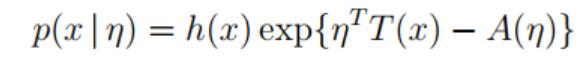其中：η：η为自然参数(natural parameter)，可以是向量形式T(x)：T(x)为充分统计量(sufficient statistic)A(η)：A(η)为累计函数(cumulant function)，作用是确保概率和为1h(x)：h(x)为underlying measure典型指数族分布转化1、Poisson分布泊松分布的标准形式为：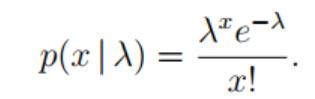其对应的指数分布形式：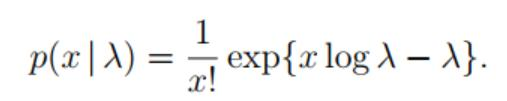其中：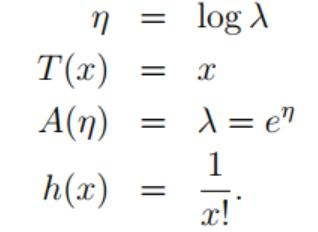2、Gaussian分布标准形式：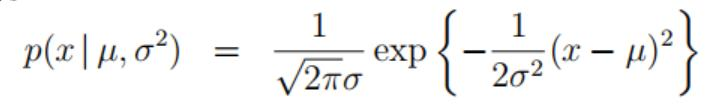指数族分布形式：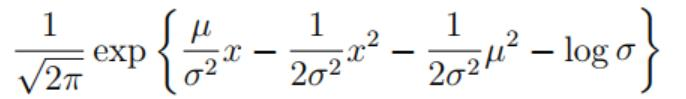其中：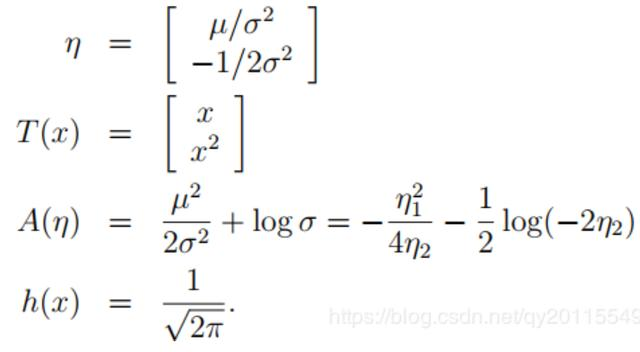3、Bernoulli分布标准形式：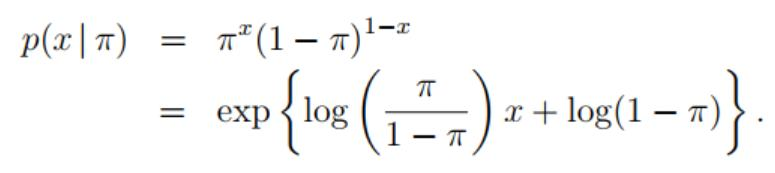其中：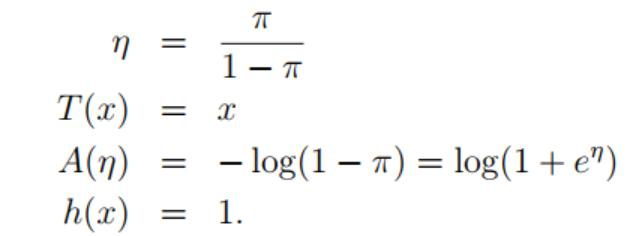4、多元Gaussian分布标准形式：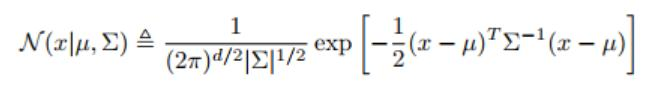指数族形式：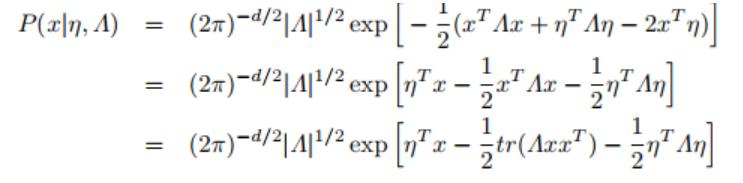5、Multinomial分布标准形式：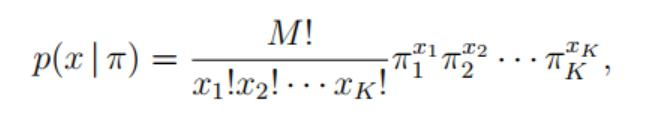指数族形式：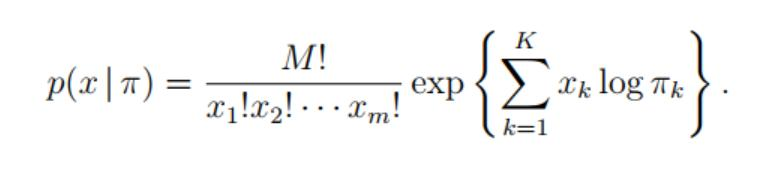总结在很多机器学习算法中，指数族分布是一个很重要的概念，比如在LDA算法，因此了解机器学习就从指数族分布开始吧。今天是三八妇女节，祝所有的妇女同胞们节日快乐！
展开全文• 指数族是一类分布，包括高斯分布、伯努利分布、二项分布、泊松分布、Beta 分布、Dirichlet 分布、Gamma 分布等一系列分布。指数族分布可以写为统一形式： p(x∣η)=h(x)exp⁡(ηTϕ(x)−A(η))=1exp⁡(A(η))h(x)...
指数族分布
指数族是一类分布，包括高斯分布、伯努利分布、二项分布、泊松分布、Beta 分布、Dirichlet 分布、Gamma 分布等一系列分布。指数族分布可以写为统一的形式：
$p(x|\eta)=h(x)\exp(\eta^T\phi(x)-A(\eta))=\frac{1}{\exp(A(\eta))}h(x)\exp(\eta^T\phi(x))$
其中，$\eta$ 是参数向量，$A(\eta)$ 是对数配分函数（归一化因子）。
在这个式子中，$\phi(x)$ 叫做充分统计量，包含样本集合所有的信息，例如高斯分布中的均值和方差。充分统计量在在线学习中有应用，对于一个数据集，只需要记录样本的充分统计量即可。
对于一个模型分布假设（似然），那么我们在求解中，常常需要寻找一个共轭先验，使得先验与后验的形式相同，例如选取似然是二项分布，可取先验是 Beta 分布，那么后验也是 Beta 分布。指数族分布常常具有共轭的性质，于是我们在模型选择以及推断具有很大的便利。
共轭先验的性质便于计算，同时，指数族分布满足最大熵的思想（无信息先验），也就是说对于经验分布利用最大熵原理导出的分布就是指数族分布。
观察到指数族分布的表达式类似线性模型，事实上，指数族分布很自然地导出广义线性模型：
$y=f(w^Tx)\\ y|x\sim Exp Family$
在更复杂的概率图模型中，例如在无向图模型中如受限玻尔兹曼机中，指数族分布也扮演着重要作用。
在推断的算法中，例如变分推断中，指数族分布也会大大简化计算。
一维高斯分布
一维高斯分布可以写成：
$p(x|\theta)=\frac{1}{\sqrt{2\pi}\sigma}\exp(-\frac{(x-\mu)^2}{2\sigma^2})$
将这个式子改写：
$\frac{1}{\sqrt{2\pi\sigma^2}}\exp(-\frac{1}{2\sigma^2}(x^2-2\mu x+\mu^2))\\ =\exp(\log(2\pi\sigma^2)^{-1/2})\exp(-\frac{1}{2\sigma^2}\begin{pmatrix}-2\mu&1\end{pmatrix}\begin{pmatrix}x\\x^2\end{pmatrix}-\frac{\mu^2}{2\sigma^2})$
所以：
$\eta=\begin{pmatrix}\frac{\mu}{\sigma^2}\\-\frac{1}{2\sigma^2}\end{pmatrix}=\begin{pmatrix}\eta_1\\\eta_2\end{pmatrix}$
于是 $A(\eta)$：
$A(\eta)=-\frac{\eta_1^2}{4\eta_2}+\frac{1}{2}\log(-\frac{\pi}{\eta_2})$
充分统计量和对数配分函数的关系
对概率密度函数求积分：
KaTeX parse error: No such environment: align at position 8:
\begin{̲a̲l̲i̲g̲n̲}̲
\exp(A(\eta))&…
两边对参数求导：
$\exp(A(\eta))A'(\eta)=\int h(x)\exp(\eta^T\phi(x))\phi(x)dx\\ \Longrightarrow A'(\eta)=\mathbb{E}_{p(x|\eta)}[\phi(x)]$
类似的：
$A''(\eta)=Var_{p(x|\eta)}[\phi(x)]$
由于方差为正，于是 $A(\eta)$ 一定是凸函数。
充分统计量和极大似然估计
对于独立全同采样得到的数据集 $\mathcal{D}=\{x_1,x_2,\cdots,x_N\}$。
\begin{align}\eta_{MLE}&=\mathop{argmax}\eta\sum\limits{i=1}^N\log p(x_i|\eta)\nonumber\ &=\mathop{argmax}\eta\sum\limits{i=1}N(\etaT\phi(x_i)-A(\eta))\nonumber\ &\Longrightarrow A’(\eta_{MLE})=\frac{1}{N}\sum\limits_{i=1}^N\phi(x_i) \end{align}
由此可以看到，为了估算参数，只需要知道充分统计量就可以了。
最大熵
信息熵记为：
$Entropy=\int-p(x)\log(p(x))dx$

一般地，对于完全随机的变量（等可能），信息熵最大。
我们的假设为最大熵原则，假设数据是离散分布的，$k$ 个特征的概率分别为 $p_k$，最大熵原理可以表述为：
$\max\{H(p)\}=\min\{\sum\limits_{k=1}^Kp_k\log p_k\}\ s.t.\ \sum\limits_{k=1}^Kp_k=1$
利用 Lagrange 乘子法：
$L(p,\lambda)=\sum\limits_{k=1}^Kp_k\log p_k+\lambda(1-\sum\limits_{k=1}^Kp_k)$
于是可得：
$p_1=p_2=\cdots=p_K=\frac{1}{K}$
因此等可能的情况熵最大。

一个数据集 $\mathcal{D}$，在这个数据集上的经验分布为 $\hat{p}(x)=\frac{Count(x)}{N}$，实际不可能满足所有的经验概率相同，于是在上面的最大熵原理中还需要加入这个经验分布的约束。
对任意一个函数，经验分布的经验期望可以求得为：
KaTeX parse error: Got function '\hat' with no arguments as subscript at position 13:
\mathbb{E}_\̲h̲a̲t̲{p}[f(x)]=\Delt…
于是：
$\max\{H(p)\}=\min\{\sum\limits_{k=1}^Np_k\log p_k\}\ s.t.\ \sum\limits_{k=1}^Np_k=1,\mathbb{E}_p[f(x)]=\Delta$
Lagrange 函数为：
$L(p,\lambda_0,\lambda)=\sum\limits_{k=1}^Np_k\log p_k+\lambda_0(1-\sum\limits_{k=1}^Np_k)+\lambda^T(\Delta-\mathbb{E}_p[f(x)])$
求导得到：
$\frac{\partial}{\partial p(x)}L=\sum\limits_{k=1}^N(\log p(x)+1)-\sum\limits_{k=1}^N\lambda_0-\sum\limits_{k=1}^N\lambda^Tf(x)\\ \Longrightarrow\sum\limits_{k=1}^N\log p(x)+1-\lambda_0-\lambda^Tf(x)=0$
由于数据集是任意的，对数据集求和也意味着求和项里面的每一项都是0：
$p(x)=\exp(\lambda^Tf(x)+\lambda_0-1)$
这就是指数族分布。


展开全文机器学习 算法
• 指数分布族是一系列分布的统称，包含连续和离散的相关分布。例如，正态分布(Gaussian)、泊松分布（Poisson）、二项分布(Bernoulli)、指数分布(exponential)、Gamma分布、多项式分布(multivariate)等。指数分布族中的...
• 第二章Probability Distributions贝塔-二项式、狄利克雷-多项式共轭、高斯分布指数族等很基础也很重要。
• 亦称指数分布族，是统计中最重要参数分布族，包含了二项分布、正态分布、泊松分布等。 概率密度函数可以表达为如下形式： 如果a(y)=y，则指数族分布为标准形式（Standard Form），b(θ)称为自然参数...python
• 指数族是一类分布，包括高斯分布、伯努利分布、二项分布、泊松分布、Beta 分布、Dirichlet 分布、Gamma 分布等一系列分布。指数族分布可以写为统一形式： p(x∣η)=h(x)exp⁡(ηTϕ(x)−A(η))=1exp⁡(A(η))h(x)...机器学习 统计学
• 指数族分布有：高斯分布、伯努利分布、二项分布、泊松分布、beta分布、Dirichlet分布、gamma分布等 指数族分布有6大性质： 充分统计量 共轭 最大熵 广义线性模型 概率图模型 变分推断 指数族分布的...
• 主讲人 网络上尼采 （新浪微博: @Nietzsche_复杂网络机器学习） 网络上尼采(813394698)9:11:56 开始吧，先不要发言了，先讲PRML第章Probability... 顾名思义，PRML第章ProbabilityDistribut...
• 一、介绍一般形式指数族分布有：高斯分布、伯努利分布、二项分布、泊松分布、beta分布、Dirichlet分布、gamma分布等。指数族分布的一般形式： 其中：① ：参数向量；② ：充分统计量，Sufficient statistic；③ ：...
• 指数族分布有：高斯分布、伯努利分布、二项分布、泊松分布、beta分布、Dirichlet分布、gamma分布等。 指数族分布的一般形式： P(x∣η)=h(x)exp{ηTϕ(x)−A(η)}P(x|\eta )=h(x)exp\left \{\eta ^{T}\phi (x)-A(\...机器学习 算法 人工智能 数据挖掘
• 一、介绍一般形式指数族分布有：高斯分布、伯努利分布、二项分布、泊松分布、beta分布、Dirichlet分布、gamma分布等。指数族分布的一般形式：其中：①：参数向量；②：充分统计量，Sufficient statistic；③：log ...
• 指数族分布有：高斯分布、伯努利分布、二项分布、泊松分布、beta分布、Dirichlet分布、gamma分布等。 指数族分布的一般形式： P(x∣η)=h(x)exp{ηTϕ(x)−A(η)}P(x|\eta )=h(x)exp\left \{\eta ^{T}\phi (x)-A(\...机器学习
• 一、Point：指数族分布的形式以及偏导的形式（1）指数族分布的形式指数族分布指的不是某个具体的分布，而是一种抽象形式，可以具象化为高斯分布，伯努利分布，二项分布，Beta分布以及狄利克雷...
• 对于二项分布η(p)=log⁡p1−p,A(η)=nlog⁡(1+eη)\eta(p) = \log\frac{p}{1-p},A(\eta)=n\log(1+e^{\eta})η(p)=log1−pp​,A(η)=nlog(1+eη) 首先有p=eη1+eηp = \frac{e^{\eta}}{1+e^{\eta}}p=1+eηeη​ A′...
• 指数族是概率分布的重要一类，包括正态分布，伽玛分布，β分布，指数分布，泊松分布，二项分布和伯努利分布。 有关此库背后基本概念的说明，请参见我们的。 框架 表示 EFAX的对象有一个单一的基类：对分布族和分布...Python
• 标签： 机器学习概率分布这章主要介绍...密度估计⎧⎩⎨⎪⎪⎪⎪参数方法⎧⎩⎨⎪⎪给出分布形式，通过最大似然、最大后验等调整参数大小（多为指数族分布） 离散变量：二项式、多项式分布 连续变量：Gauss分布非参机器学习
• 1. 伯努利分布以和二项分布 考虑随机变量，对应抛一枚硬币（不一定均匀），当硬币正面朝上取1, 反面朝上取0。如果 x = 1 概率记为，则，很明显，所以概率分布可以表示为： 我们把这种分布称为伯努利分布。易证...
• 在讲义的第一部分中，Ng首先讲解了什么叫做监督学习，其次讲了用最小二乘法求解的线性模型，用sigmod函数表示响应函数的logistics回归，接着，利用这两种模型，推出了一种应用十分广泛的指数分布族，在指数分布族的...函数 机器学习 广义线性模型
• 提到广义线性模型(GLM)可概括为服务于一组来自指数分布族的响应变量模型框架，正态分布、指数分布、伽马分布、卡方分布、贝塔分布、伯努利分布、二项分布、多项分布、泊松分布、负二项分布、集合分布等都属于指数...
• 1、线性模型假设Y是正态分布，Y的条件均值是β的线性函数 2、指数分布族假定Yi来自指数分布族，常见的正态分布、二项分布、泊松分布、伽马分布都是指数分布族指数分布族的概率密度： 注：泊松分布和二项分布的φ=13...
• 指数族分布(exponential family of distributions)亦称指数分布族，在上世纪30年代中期被提出,在概率学和统计学中,它是一些有着特殊形式的概率分布的集合,是统计中最重要的参数分布族，包含了二项分布、正态分布,...Logistic回归 逻辑回归
• Logstic回归采用sigmoid函数原因（sigmoid函数能表示二项分布概率原因） sigmoid函数： $f(x)=\frac{1}{1+e^{-x}}$ 直觉上，采用sigmoid函数来模拟(0, 1)段函数是...将二项分布表示成指数族分布： \[\begin...
• 提到广义线性模型(GLM)可概括为服务于一组来自指数分布族的响应变量模型框架，正态分布、指数分布、伽马分布、卡方分布、贝塔分布、伯努利分布、二项分布、负二项分布、多项分布、泊松分布、集合分布等都属于指数...r语言线性回归r语言
• 分布1）两点分布2）二项分布3）泊松分布4）均匀分布5）指数分布6）正态分布7）前几个分布总结8）Beta分布9）指数伯努利分布属于指数高斯分布也属于指数10）Sigmoid/Logistic函数二、统计量1.事件独立性2....机器学习
• 分布1）两点分布2）二项分布3）泊松分布4）均匀分布5）指数分布6）正态分布7）前几个分布总结8）Beta分布9）指数伯努利分布属于指数高斯分布也属于指数10）Sigmoid/Logistic函数二、统计量1.事件独立性2....